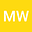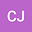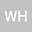Enhanced Language Model with Hybrid Knowledge Graph for Mathematical Topic Prediction
•••• +1Canghong Jin
Zhejiang University City College
Author ProfileWenkang Hu
Zhejiang University College of Computer Science and Technology
Author ProfileYabo Chen
Zhejiang University
Author Profile## Abstract

Understanding mathematical topics is important for both educators and students to capture latent concepts of questions, evaluate study performance, and recommend content in online learning systems. Compared to traditional text classification, mathematical topic classification has several main challenges: (1) the length of mathematical questions is relatively short; (2) there are various representations of the same mathematical concept(i.e., calculations and application); (3) the content of question is complex including algebra, geometry, and calculus. In order to overcome these problems, we propose a framework that combines content tokens and mathematical knowledge concepts in whole procedures. We embed entities from mathematics knowledge graphs, integrate entities into tokens in a masked language model, set up semantic similarity-based tasks for next-sentence prediction, and fuse knowledge vectors and token vectors during the fine-tuning procedure. We also build a Chinese mathematical topic prediction dataset consisting of more than 70,000 mathematical questions with topics. Our experiments using real data demonstrate that our knowledge graph-based mathematical topic prediction model outperforms other state-of-the-art methods.